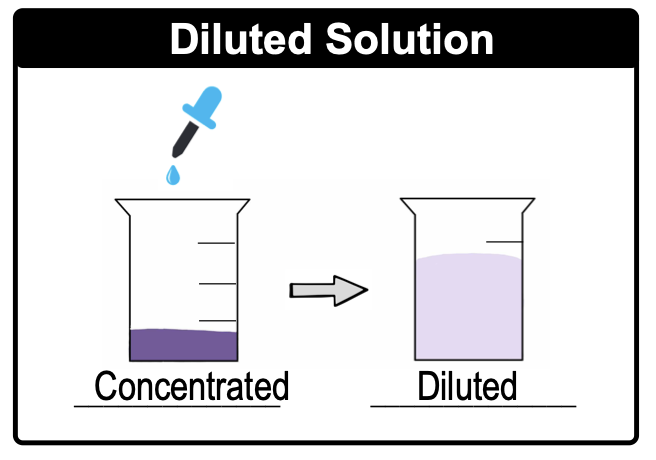Clutch Prep is now a part of Pearson

# Dilutions

See all sections
Sections
Solutions
Solubility and Intermolecular Forces
Solutions: Mass Percent
Percent Concentrations
Molarity
Osmolarity
Parts per Million (ppm)
Solubility: Temperature Effect
Intro to Henry's Law
Henry's Law Calculations
Dilutions
Solution Stoichiometry
Electrolytes (Simplified)
Equivalents
Molality
The Colligative Properties
Boiling Point Elevation
Freezing Point Depression
Osmosis
Osmotic Pressure

In Dilutions, a solvent (usually water) is added to a concentrated solution.

###### Concentrated & Diluted Solutions

Concept #1: DilutionsExample #1: If each sphere represents a mole of solute from the images provided below, arrange the solutions from least concentrated to most concentrated.

Concept #2: Dilution CalculationsExample #2: What volume (in mL) of 5.2 M HBr must be used to prepare 3.5 L of 2.7 M HBr?

Practice: To what final volume would 100 mL of 5.0 M KCl have to be diluted in order to make a solution that is 0.54 M KCl?

Practice: If 880 mL of water is added to 125.0 mL of a 0.770 M HBrO4 solution what is the resulting molarity?

Practice: A student prepared a stock solution by dissolving 25.00 g of NaOH in enough water to make 150.0 mL solution. The student took 20.0 mL of the stock solution and diluted it with enough water to make 250.0 mL solution. Finally taking 75.0 mL of that solution and dissolving it in water to make 500 mL solution. What is the concentration of NaOH for this final solution? (MW of NaOH: 40.00 g/mol).